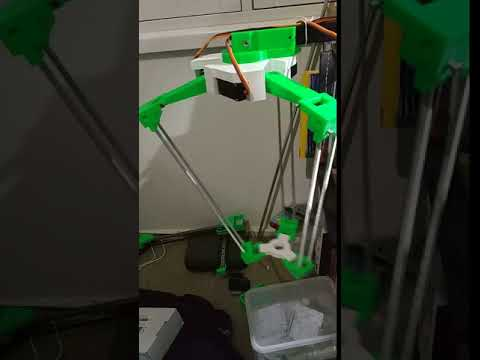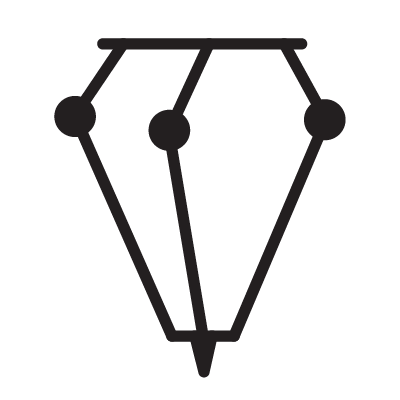# Delta-Kinematics-Library

https://github.com/tinkersprojects/Delta-Kinematics-Library# Delta-Kinematics-Library

Forward and Inverse Kinematics Library for Delta robot.

Thank you to:

R.L. Williams II, “The Delta Parallel Robot: Kinematics Solutions”, Internet Publication, www.ohio.edu/people/williar4/html/pdf/DeltaKin.pdf, January 2016.

## Functions

### SETUP

#### DeltaInverseKinematics(double *angleB1,double *angleB2,double *angleB3,double ArmLength,double RodLength,double BassTri,double PlatformTri);

This Function is set up the variables that will be returning for the motors. ArmLength, RodLength, BassTri and PlatformTri are the set values from the Delta robot.

### SET

#### void set(double x,double y,double z);

This function to calculate the angle of each motor given the position X, Y and Z. The motor angles will be returned through the variables declared in the setup;angleB1, angleB2 and angleB3. the values of the angles are in radians.

#### void setOffsets(double X, double Y, double Z);

This function is used to set the Offsets of X, Y and Z.

#### void setLimits(double upperX, double upperY, double upperZ, double lowerX, double lowerY, double lowerZ);

This function is used to set the angle limits of each motor.